Covariances for the LANL $^{239}$Pu cross section evaluationLA-UR-22-31089

### Matthew Mumpower

on behalf of the LANL teamNuclear Data TeamLos Alamos National Laboratory Caveat

The submitted materials have been authored by an employee or employees of Triad National Security, LLC (Triad) under contract with the U.S. Department of Energy/National Nuclear Security Administration (DOE/NNSA).

Accordingly, the U.S. Government retains an irrevocable, nonexclusive, royaltyfree license to publish, translate, reproduce, use, or dispose of the published form of the work and to authorize others to do the same for U.S. Government purposes.

## Methodology

We are in the process of updating the $^{239}$Pu cross sections in the fast energy range
(complementing the work of IAEA / INDEN / ORNL / LLNL)

Covariances constructed with NEXUS KALMAN code

Method: Combine model variation with experimental uncertainties

Bayesian inference used to estimate covariances

Posterior covariances for parameters take the form:

$\pmb{P} = (\pmb{X}^{-1} + \pmb{C}^{T} \pmb{V}^{-1} \pmb{C}) ^{-1}$

And the final covariance matrix (function of energy) is:

$\pmb{F} = \pmb{C} \pmb{P} \pmb{C}^{T}$

Where:

$\pmb{X}$ = prior model parameter cov. matrix, $\pmb{V}$ = experimental data cov. matrix, $\pmb{C}$ = model sensitivity matrix

Rising et al. Nuclear Science and Engineering 175 81 (2013)

## Total cross section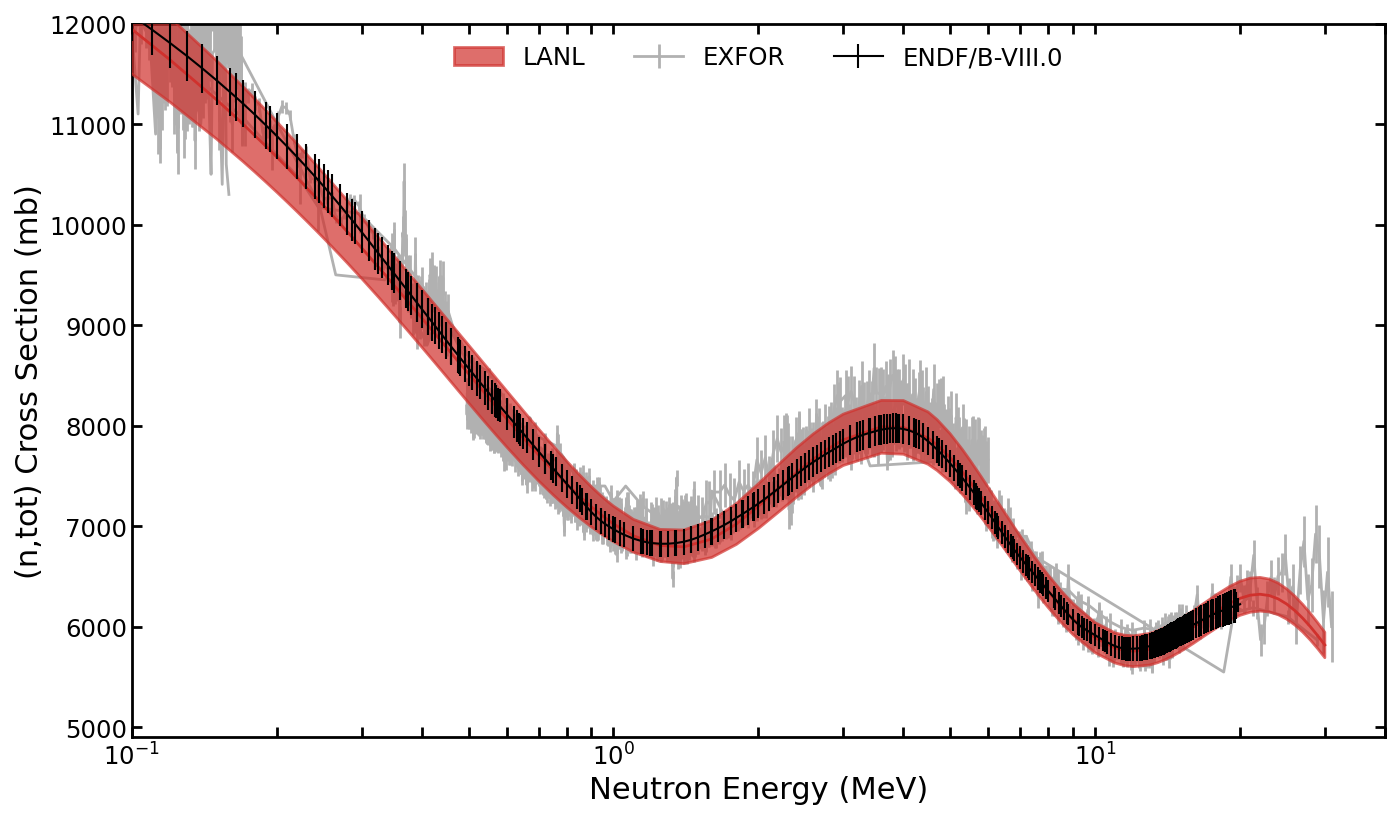Model: Soukhovitskii (2005) optical model [deformation ~0.21]

Uncertainties generated from variation of 7 optical model parameters and 25+ experimental datasets

## Total: energy correlation matrix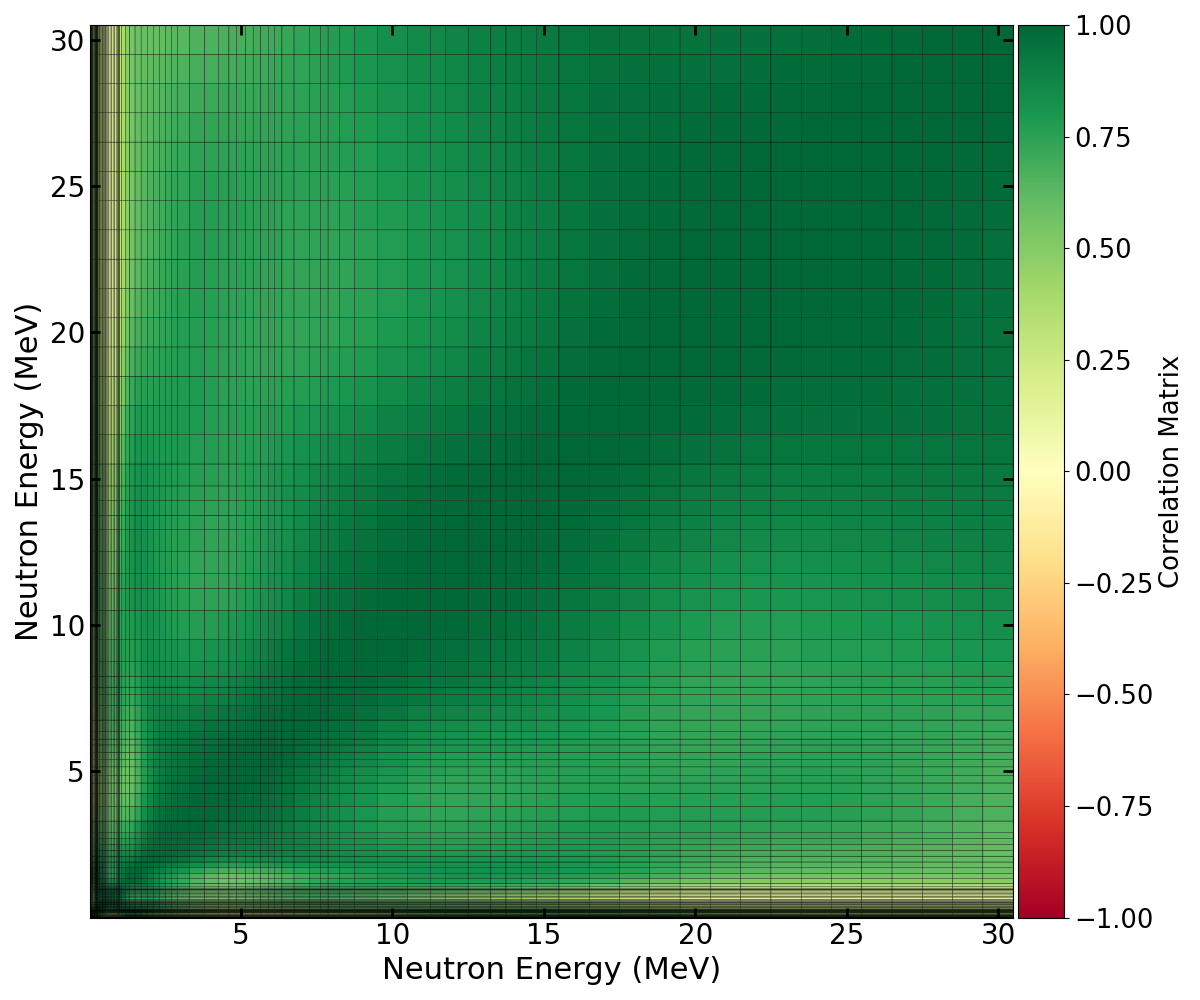Generally positive correlation as a function of energy

Assume the off-diagonal elements of the experimental covariance matrix are relatively correlated (0.3)

## Total: relative uncertainty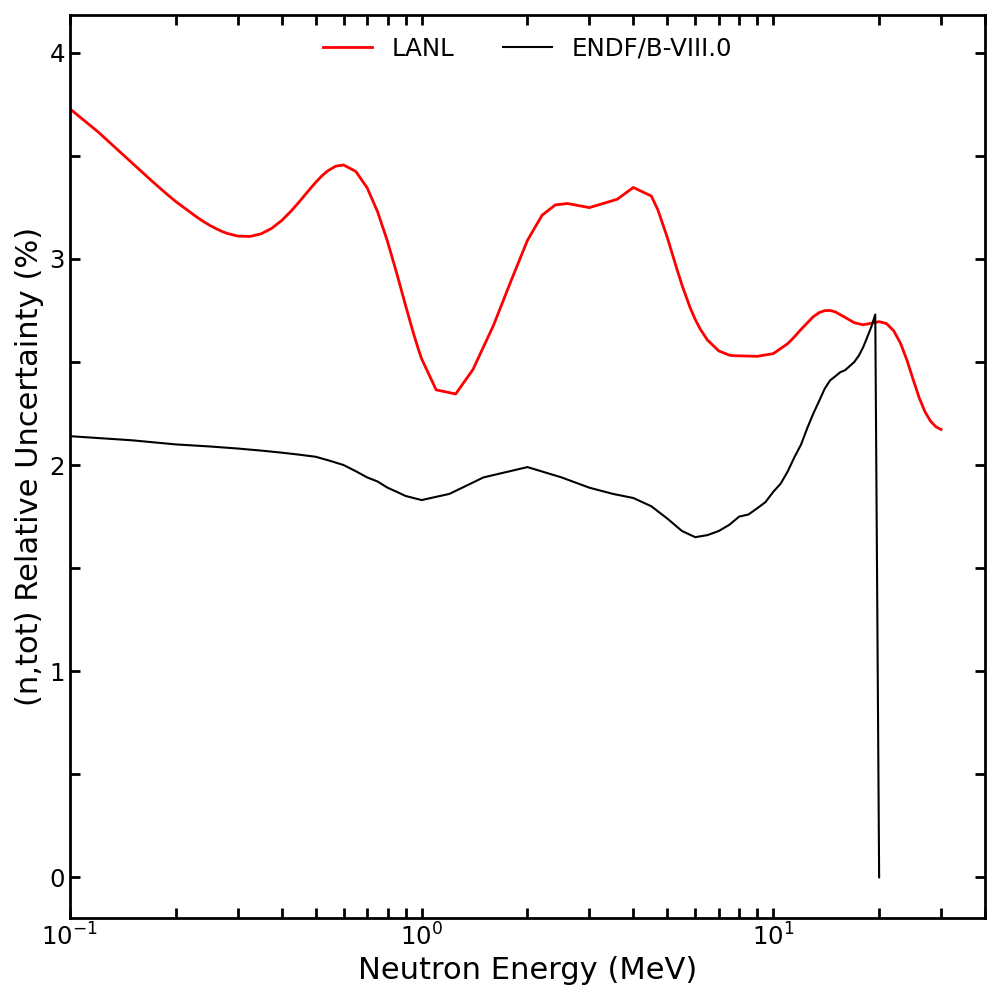LANL result is consistently higher than ENDF/B-VIII.0

Reason for differences are under analysis

## Capture cross section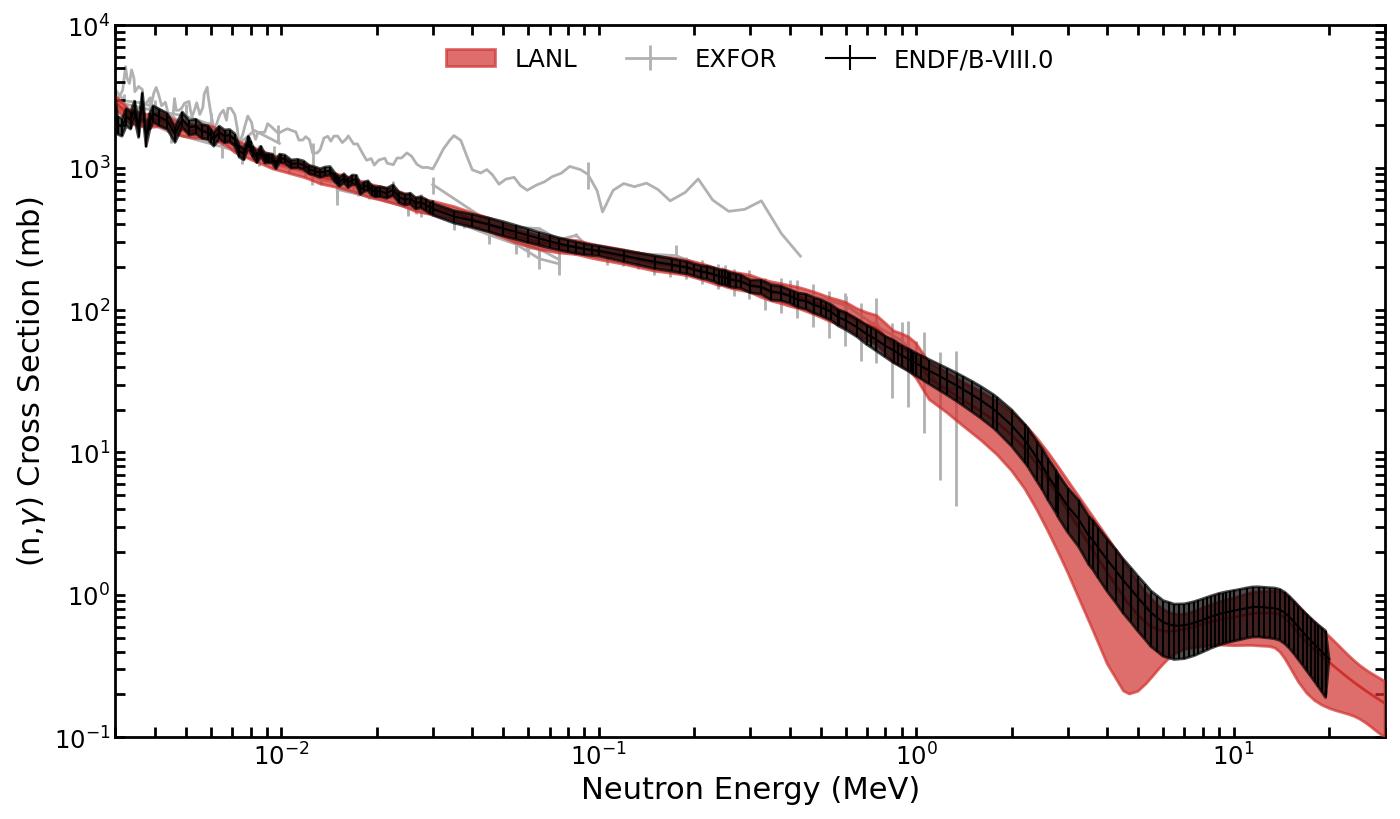Model: CoH statistical Hauser Feshbach with M1 of Mumpower et al. PRC 96 024612 (2017)

Uncertainties generated from 20+ model parameters and 10+ experimental datasets

## Capture: energy correlation matrix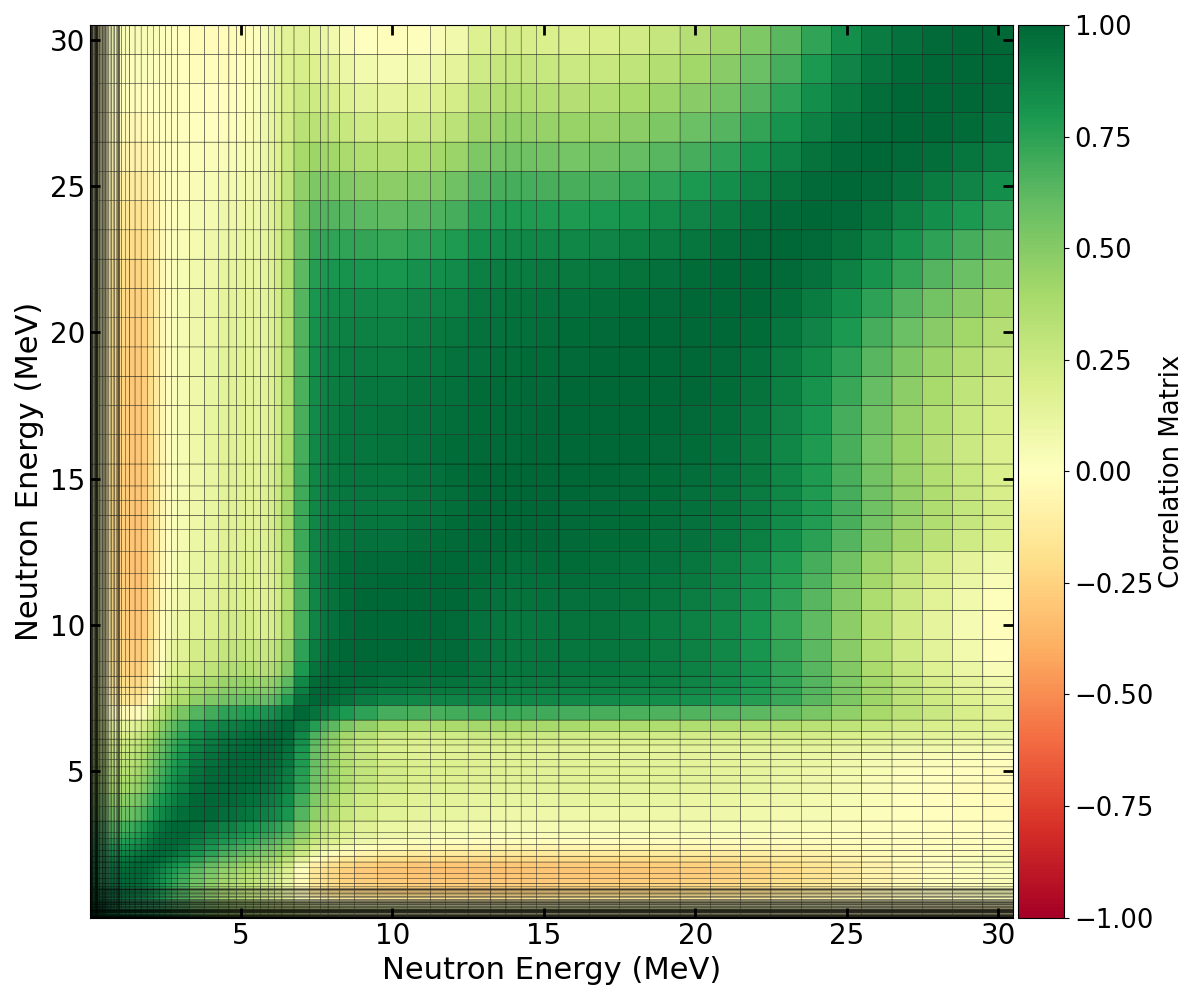Diagonal or near diagonal positive correlation; off-diagonal negative as a function of energy

Assume the off-diagonal elements of the experimental covariance matrix are relatively correlated (0.3)

## Capture: relative uncertainty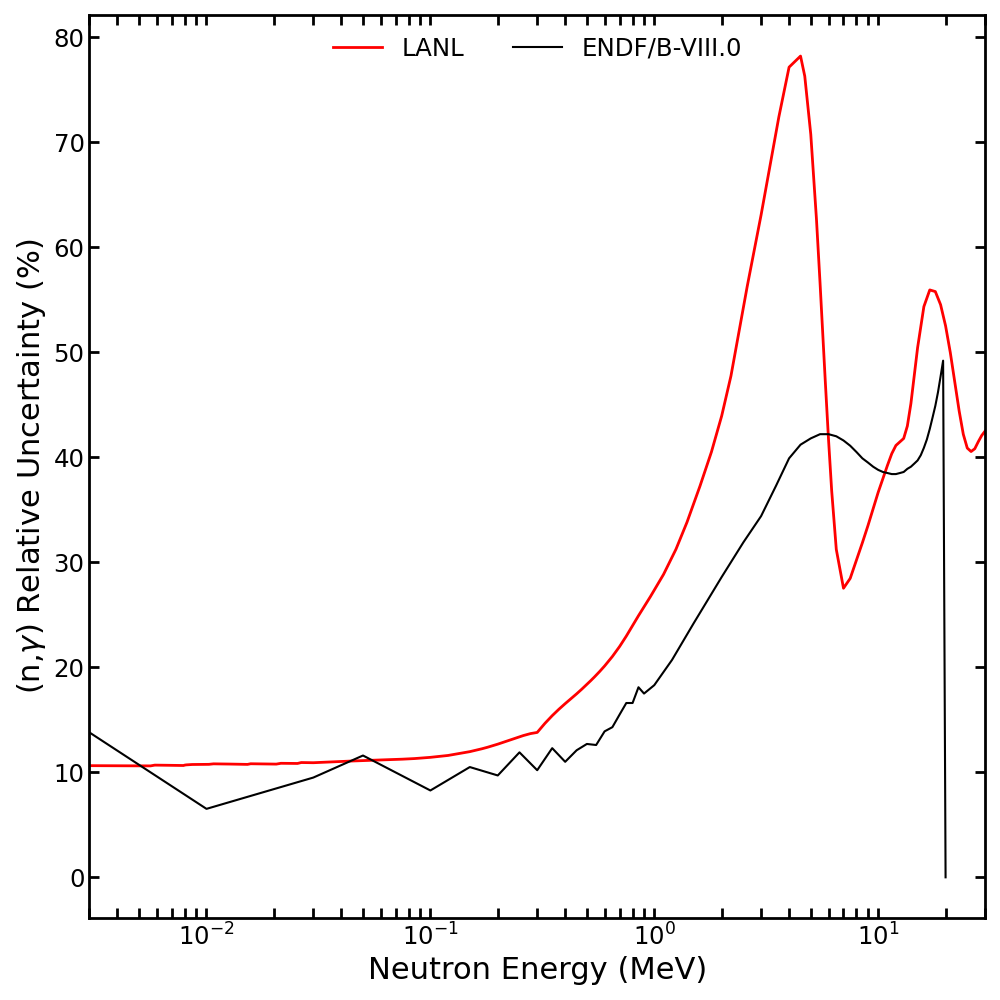LANL result is consistently higher at higher energies than ENDF/B-VIII.0

New Mosby (2018) data is most impactful to new uncertainties

## (n,2n) cross section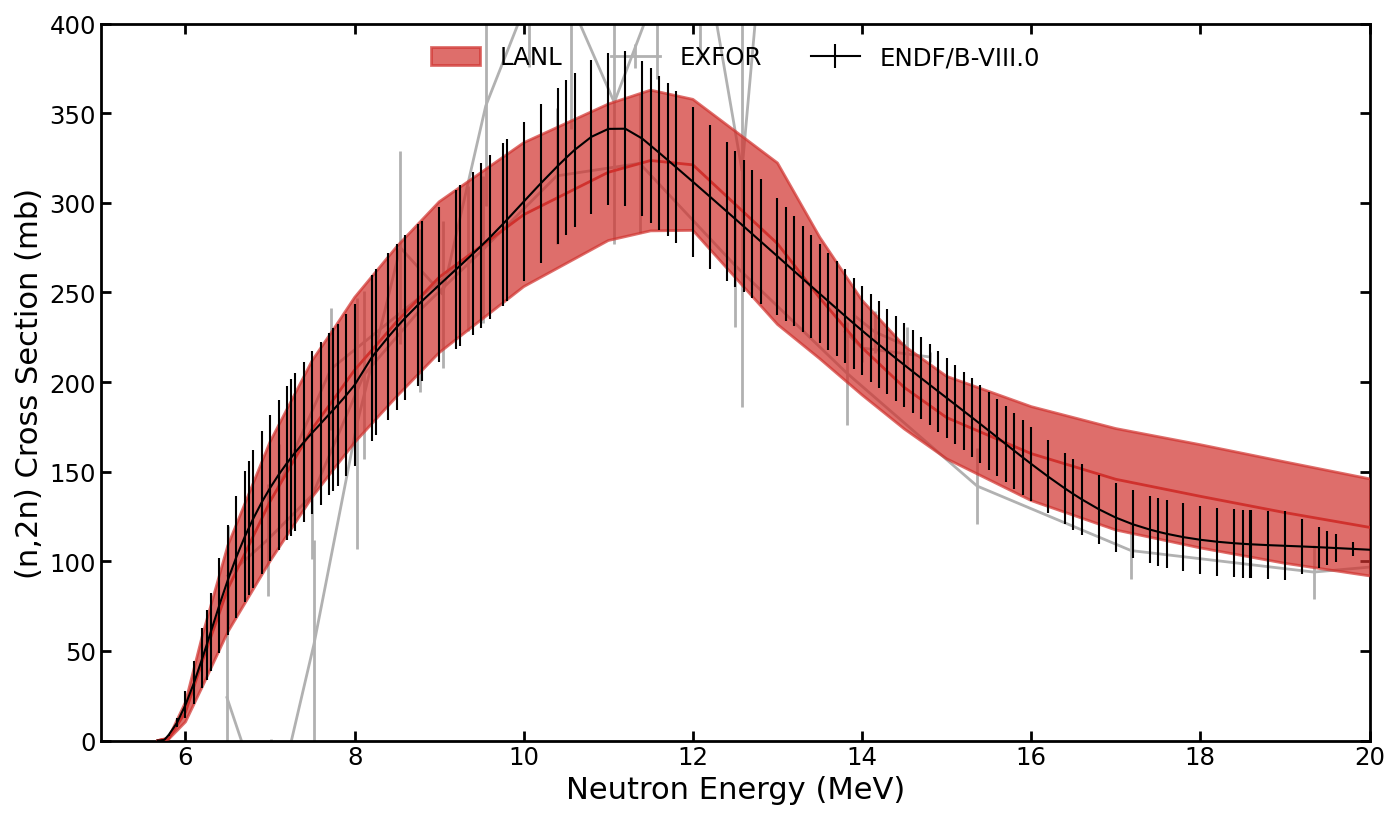Model: CoH with new collective enhancement of Mumpower et al. submitted PRC (2022)

Uncertainties generated from variation of 20+ model parameters and 4 experimental datasets

## (n,2n): energy correlation matrix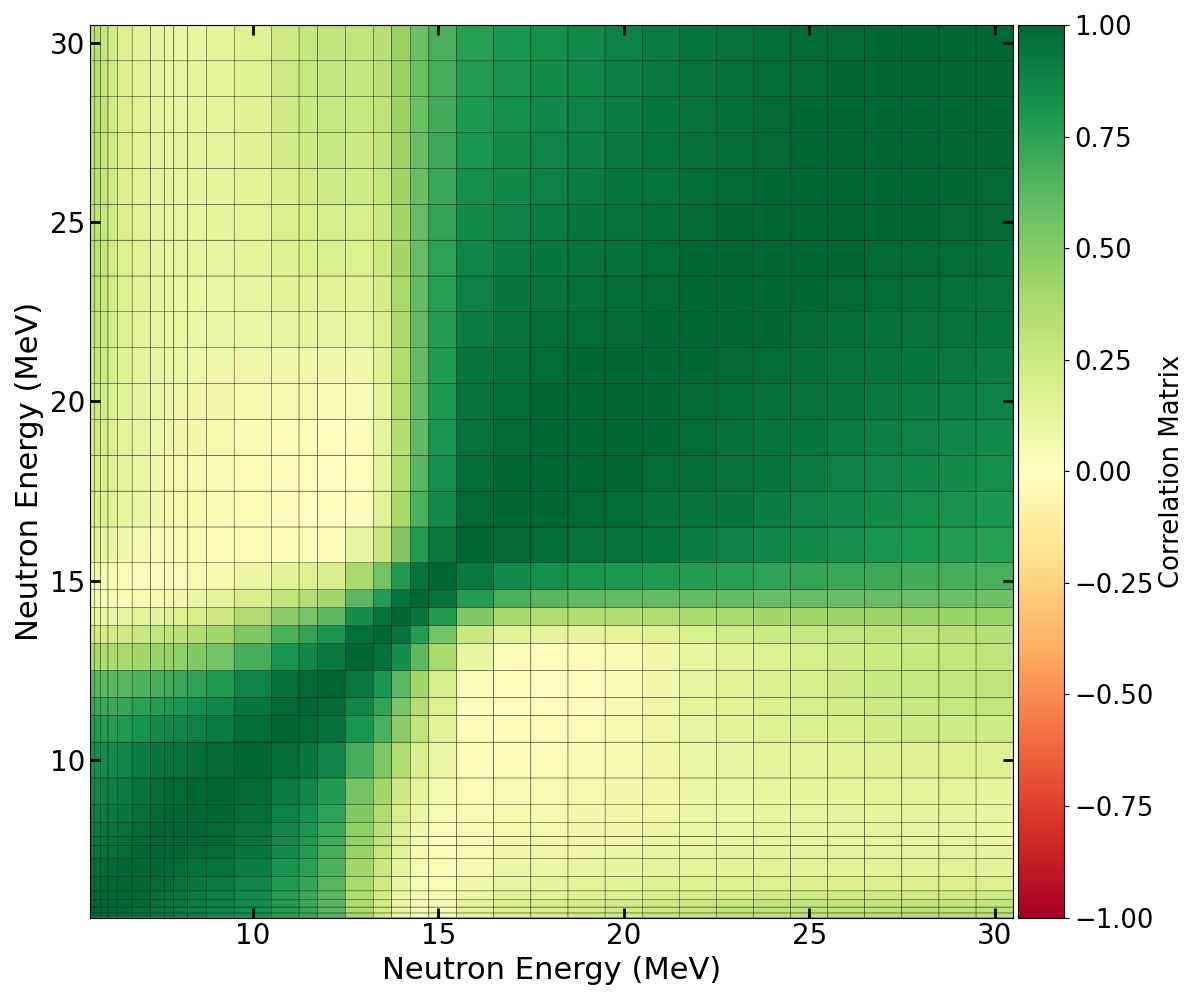Generally positive correlation as a function of energy

Assume the off-diagonal elements of the experimental covariance matrix are relatively correlated (0.3)

## (n,2n): relative uncertainty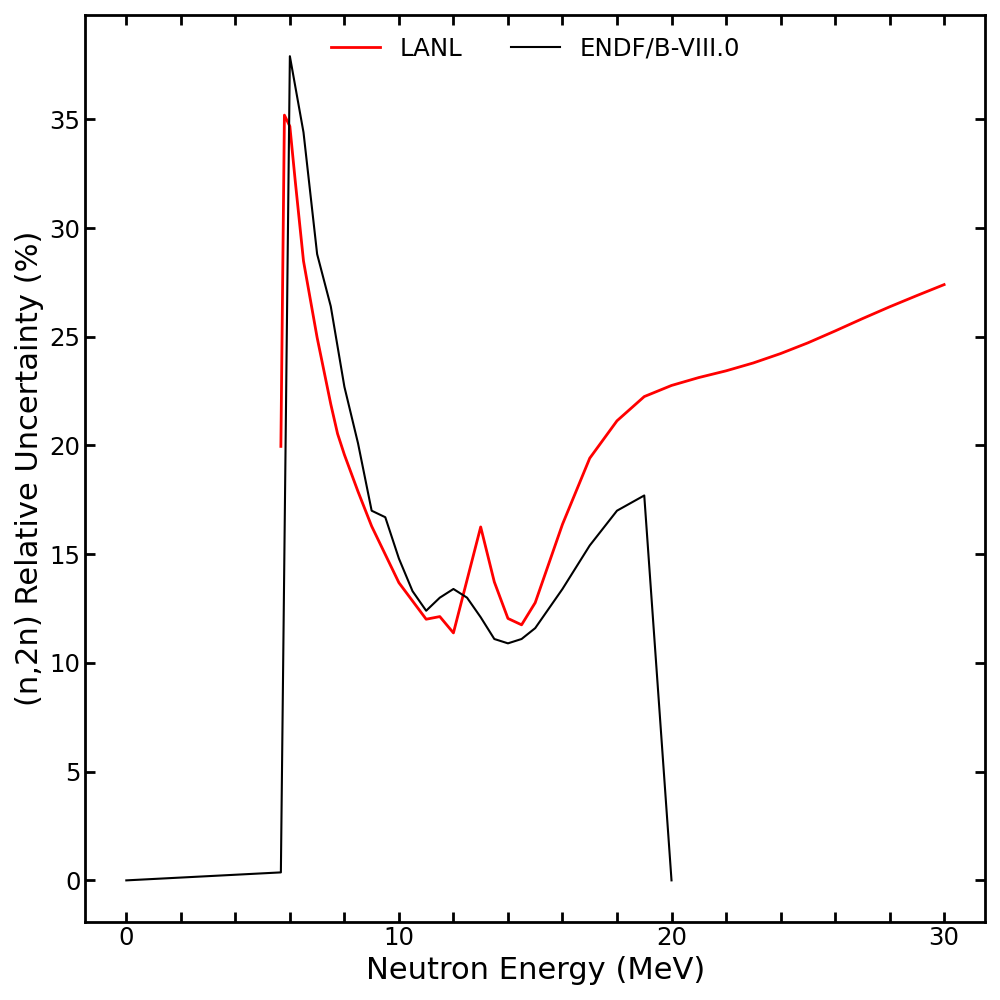LANL result is consistently similar order as ENDF/B-VIII.0

Differences arises from new collective enhancement and Méot et al. PRC 103 054609 (2021) data near threshold

## (n,inel) cross section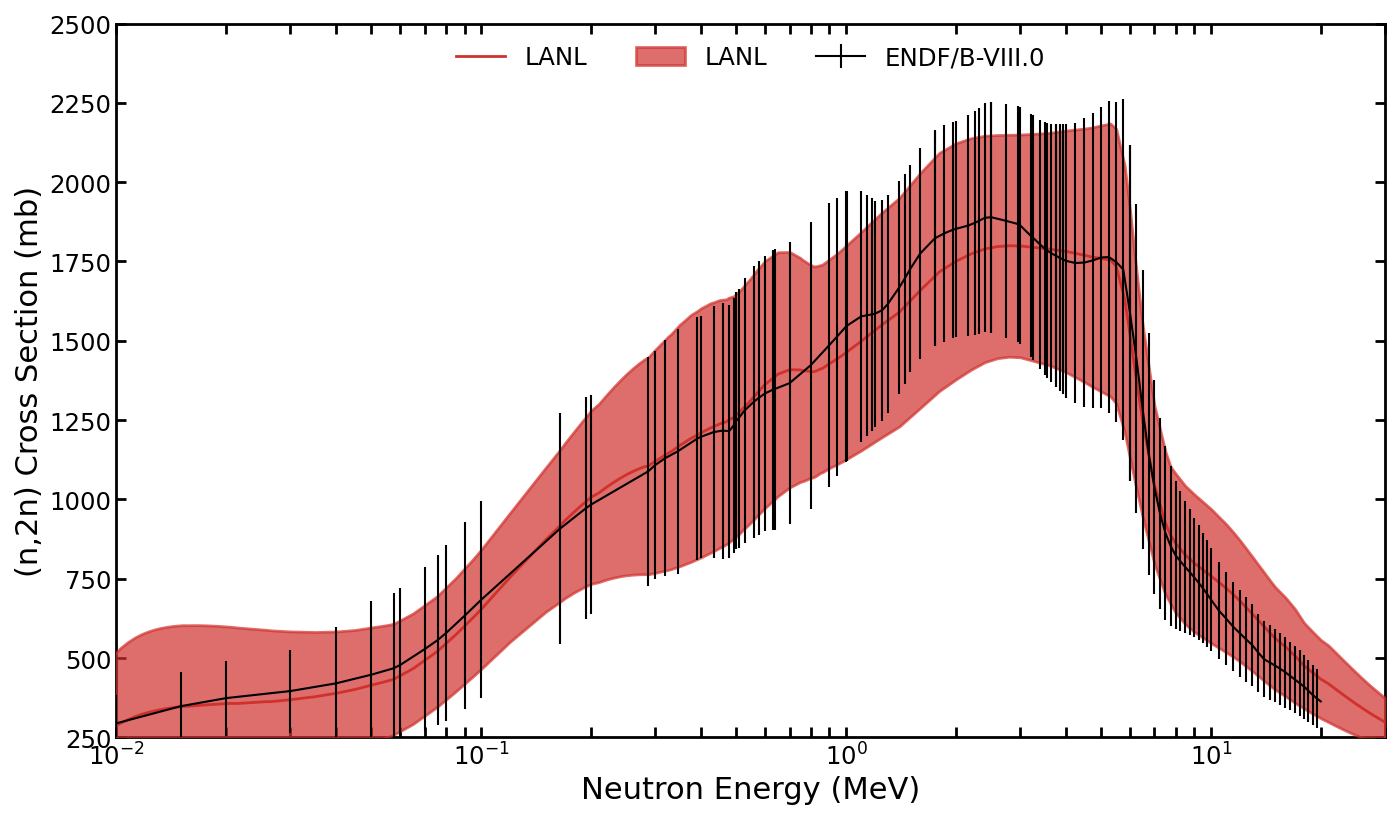Model: CoH statistical model

Uncertainties generated from variation of 20+ model parameters and 1 experimental dataset

## (n,inel): energy correlation matrix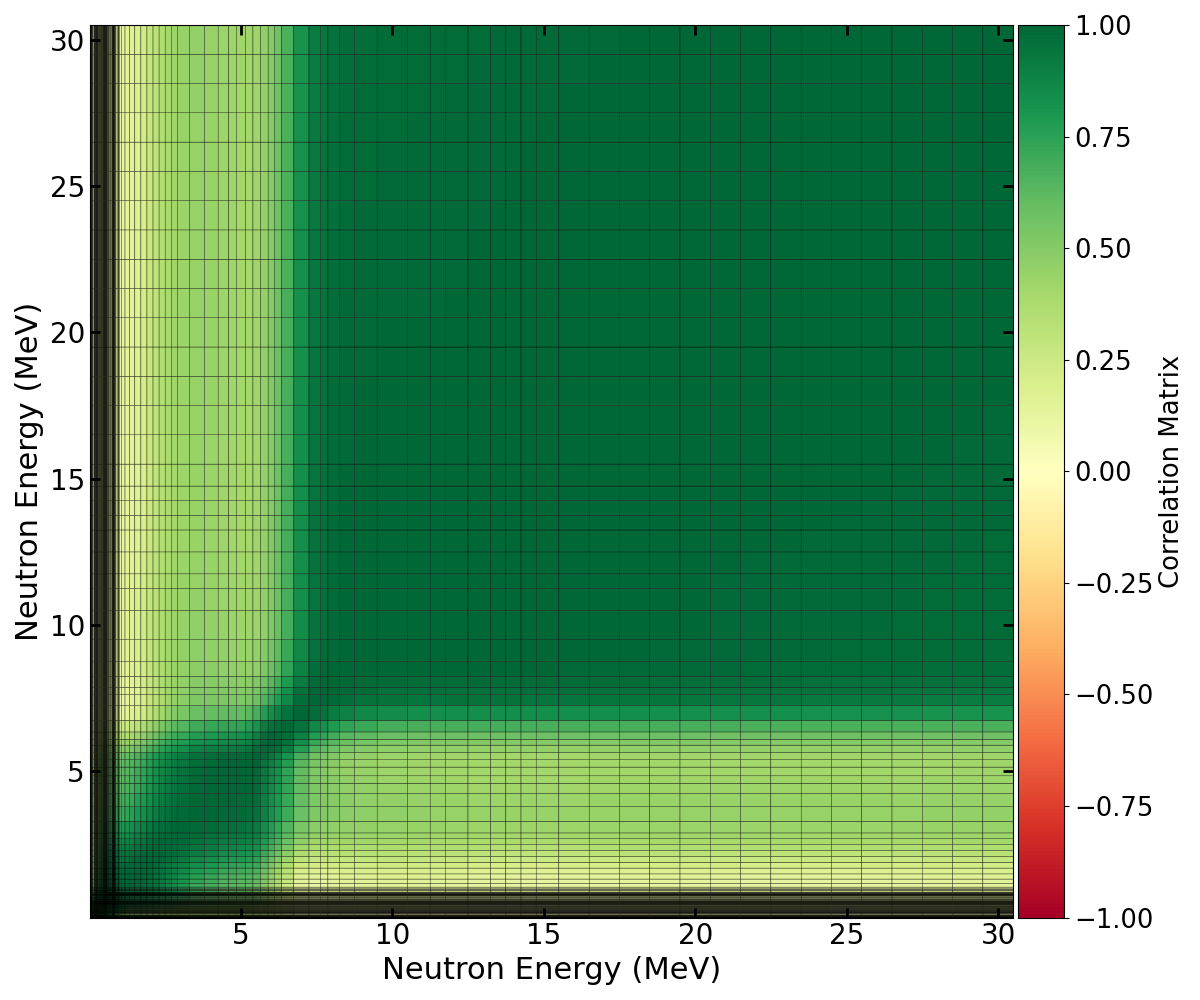Generally positive correlation as a function of energy

Assume the off-diagonal elements of the experimental covariance matrix are relatively correlated (0.3)

## (n,inel): relative uncertainty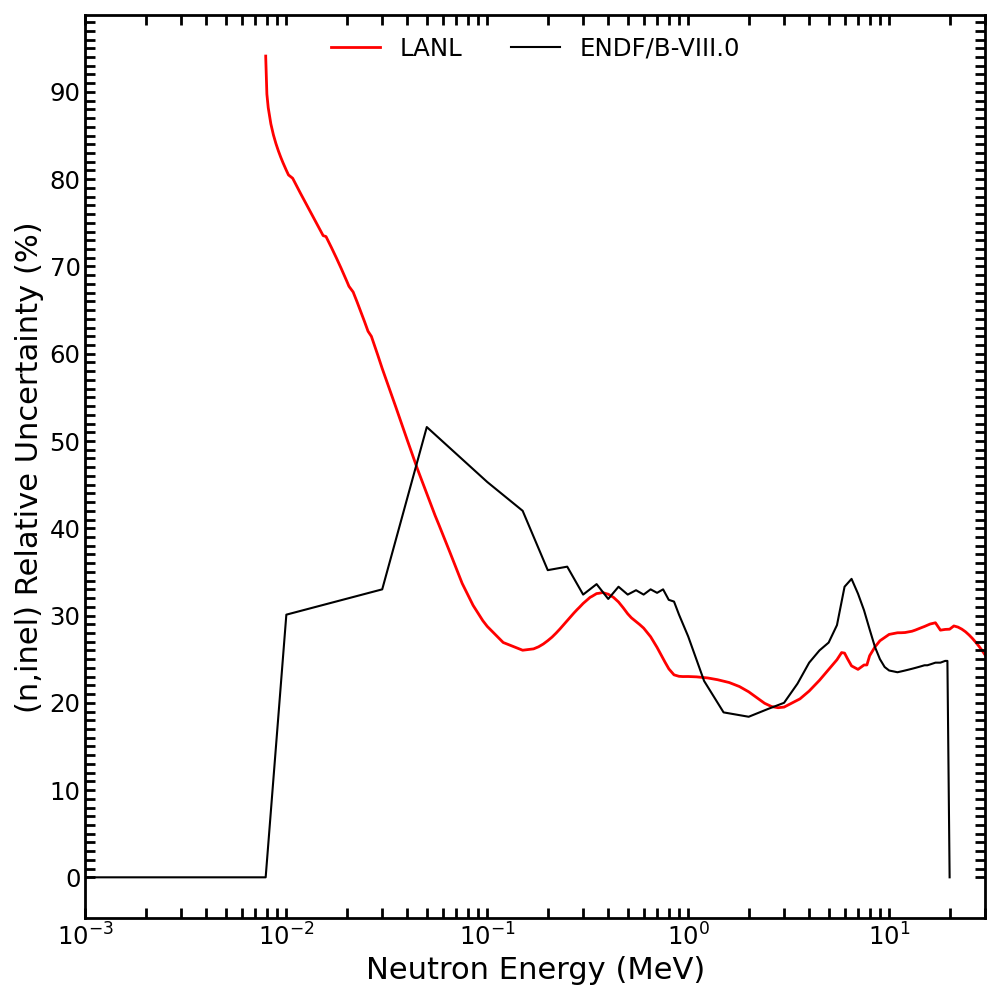LANL result is consistently similar order as ENDF/B-VIII.0

Cross section near threshold is very uncertain and model dependent

## Special thanks to our colleagues

Roberto Capote, Mark Chadwick, Matt Devlin, Nathan Gibson, Wim Haeck, Mike Herman, Toshihiko Kawano, Keegan Kelly, Noah Kleedtke, Amy Lovell, Paola Marini, Denise Neudecker, Vladimir Pronyaev, Hiro Sasaki, Luke Snyder, Ionel Stetcu, Julien Taieb, Patrick Talou, Andrej Trkov

We gratefully acknowledge the support of the Advanced Simulation and Computing (ASC) program at Los Alamos National Laboratory.